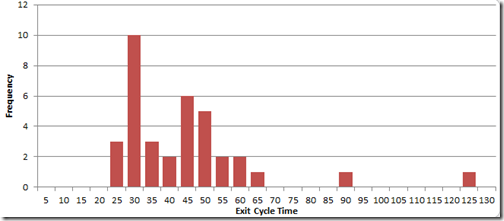# Who is Grading the Questions?

Once again the search terms have revealed an interesting test question. Let’s parse it and see what we can learn.

2. the cycle time to complete a final step on an assembly line is normally distributed, with a mean of 4 minutes and a standard deviation of 1 minute.1) at the end of an 8-hour shift, how many units will have been assembled on average?

The question is about exit cycles – the cadence at the output of a production process of some kind, in this case an assembly line.

Measuring and plotting the variation of exit cycles at the customer end of your production process is a great way to get a handle on how much variation there is.

This question, however, reveals a level of simplistic understanding in the mind of the person who wrote the question of how these things work.

First, we have to assume that this assembly line (which implies manual work) takes no breaks during their 8 hour shift. We have no information that suggests otherwise.

That means we have 8 hours x 60 minutes = 480 minutes of working time.

Intuitively, if the exit cycles are normally distributed (which implies the distribution is symmetric), with a mean of 4 minutes, then we should be able to simply divide to get the average number of units built during each shift.

480 / 4 = 120 units

Which in the long-haul is close, though it will take a lot of iterations to converge on that. Running about 65 iterations of a random number set with a mean of 4 and standard deviation of 1, I got an average daily production of 120.1, a high of 127 units, and a low of 115.

## But Exit Cycle Times Aren’t Normally Distributed

This is a problem with a lot of the “statistics” I see being kicked around today.

This is a histogram of 36 actual exit cycle times from a real production line.The “Cycle Time” bucket numbers on the horizontal axis represent the top of the range. So the high bar says there were 10 cycle times between 25 and 30 seconds.

The mean of the data set is 42, which is actually one of the less frequent values.

The first thing that jumps out is that this data set might have two peaks (be bimodal). I would want a lot more data before I drew this conclusion, however let’s assume it is for the discussion because this is quite common.

A bimodal distribution implies there are two different processes running. If we looked at a run chart of individual cycles, we would probably see one of two things:

• Clusters of longer times spaced between clusters of shorter times. This would be the case if the line is running lots or batches, then switching over.
• A repeating cadence like “short-short-short-long, short-short-short-long” which would indicate really good production leveling.

But all of that is a sidebar. The real issue I have with this “homework question” simple: Cycle times are (almost) never normally distributed.

There is a minimum time that can elapse between the completion of one unit and the completion of the next, but there is no theoretical limit on the length of delay. These distributions (almost) always have a tail to the right.

So once again, be very careful about blindly using averages (arithmetic means) unless you understand the whole story.

## The Test Question Misleads the Students

And this is my problem with questions like this on exams and homework, especially in classes that purport to be teaching how factories run. Even when using a distribution, the problems almost always default to a symmetric distribution when real world factory-related data sets are rarely symmetrically distributed.

You are teaching your students a simple solution that won’t work in the real world without explaining why.

1.Steve King says: